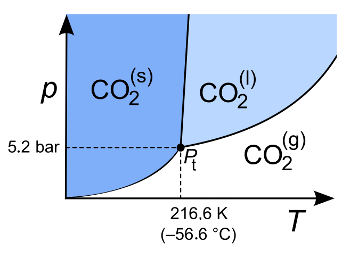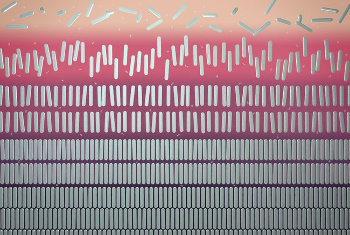### Phase Rule

November 9, 2020

Algebra was the mathematics taught during the first year of high school in my era, and it was one equation after another. The mathematics taught during the next year, Euclidean geometry (called plane geometry), was devoid of equations. Perhaps the lack of equations was the reason it was more enjoyable to me. Later, I started a course in solid geometry. This was far less enjoyable, since I disliked the teacher. Since it was not a required course, I dropped-out. If I had continued, I might have been introduced to the first important geometry equation, Euler's polyhedron formula.Euler's polyhedron formula, as written in his 1758 paper, "Elementa doctrinae solidorum" (Elements of the doctrine of solids).

The hardest part in reading this (apart from the Latin) is to remember that what looks like an "f" is actually an "s." In Euler's notation, A is the number of edges, H is the number of faces, and S is the number of vertices ("solid angles"). (Via the Scholarly Commons at the University of the Pacific. Click for larger image.)

This equation was first stated in 1758 by preeminent mathematician, Leonhard Euler (1707-1783). It describes a property of convex polyhedra based on the number of their faces (F), edges (E), and vertices (V).
V - E + F = 2
This is demonstrated for the Platonic solids in the following table.

polyhedron Verticess
V
Edges
E
Faces
F
V-E+F
Tetrahedron4 6 4 2
Cube8 12 6 2
Octahedron6 12 8 2
Dodecahedron20 30 12 2
Icosahedron12 30 20 2

Euler gave a proof for the validity of this equation; but, just as for the Pythagorean theorem, for which at least 370 unique proofs are known, many more have followed. One website gives twenty proofs of Euler's polyhedron formula.

Vertices seem to have a special role, since faces are always joined by one edge, edges join two faces, but vertices are common to a varied number of edges and faces. Thermodynamics has its own version of the vertex in the triple point, the point on the pressure-temperature phase diagram of a material at which the solid, liquid and gas phases coexist in thermodynamic equilibrium.

The triple point of water occurs at 273.1575 K (0.0075 °C, 32.0135 °F) and a partial pressure of water vapor of 6.11657 mbar. Although the pressure is much below atmospheric pressure, what's important here is the partial pressure of water; and, since the slope of the solid-liquid phase boundary is steep, the temperature is not that removed from what's considered to be the melting point of ice.Triple point of carbon dioxide in its pressure-temperature phase diagram.

You will only see liquid CO2 at very high pressures. That's why there's a transition from solid to gas (sublimation) at standard laboratory conditions.

(Wikimedia Commons image by Sponk (Modified). Click for larger image.)

The thermodynamics of phase equilibrium, as for the triple point, are summarized in the phase rule, derived by eminent thermodynamicist, J. Willard Gibbs (1839-1903), around 1875. Gibbs created statistical mechanics, which explains the laws of thermodynamics in terms of the statistical properties of ensembles of particles. This phase rule equation, reminiscent of Euler's polyhedron formula, is as follows:
F = C - P + 2

In this equation, F is the number of degrees of freedom of the system, C is the number of its components, and P is the number of phases. If we consider the triple point of a pure substance (C=1), we know that a point has zero degrees of freedom, so we get
0 = 1 -P +2

P = 3

Although Einstein apparently said that Gibbs' thermodynamics was the only theory he really trusted, researchers from the Eindhoven University of Technology and the University Paris-Saclay have discovered a system that demonstrates a five-phase equilibrium, contrary to the Gibbs' phase rule.[3-4] The system contains an isotropic fluid, nematic and smectic liquid crystals, and two solid phases.

It was found that this system has conditions for which four phases exist at the same time, and a point at which there are five coexisting phases. This quintuple point has a gas phase, two liquid crystal phases, and two solid phases existing simultaneously. Study author, Mark Vis, an assistant professor at Eindhoven, reports that "this is the first time that the famous Gibbs rule has been broken."

As often happens in research, this phenomenon was discovered accidentally, in a manner reminiscent of Dan Shechtman's discovery of quasicrystals of supposedly impossible five-fold symmetry. Two Eindhoven graduate students, Álvaro González García and Vincent Peters saw an impossible four-phase equilibrium in computer simulations of plate-shaped particles and a polymer. Further simulations with multiple shapes, such as cubes and also rods showed the same effect. Says Remco Tuinier, a professor at Eindhoven,
"With the rods, most phases turned out to be possible, we even found a five-phase equilibrium. That could also mean that even more complicated equilibria are possible, as long as you search long enough for complex different particle shapes."

Why does this material system violate the Gibbs phase rule? Gibbs' analysis did not include the shape of particles in the mixture. Liquid crystals were discovered about a decade after Gibbs derived the phase rule, and they were only understood much later. The study authors have shown that the ratio of particle length to diameter, and the diameter of particles in relation to the diameter of other particles in the colloid-polymer mixture are as important as temperature and pressure.[3-4]A five-phase thermodynamic equilibrium. Starting from the top, a gas phase with unaligned rods (isotropic phase), then a liquid phase with rods pointing in about the same direction (nematic liquid crystal), then a liquid phase with rods aligned in different layers (smectic-phase liquid crystal), and two solid phases at the bottom.

One factor leading to this configuration is an excluded volume effect in which rods are pushed towards each other by polymer chains.

(ICMS animation studio image. Click for larger image.)

As there any uses for this discovered affect? Many products, such as mayonnaise and paint, which are colloidal mixtures, and liquid crystal displays, use similar materials. Says Vis, "Most industries choose to work with a single-phase system, where there is no segregation. But if the exact transitions are clearly described, then the industry can actually use those different phases instead of avoiding them."

### References:

1. Leonhard Euler, Elementa doctrinae solidorum (Elements of the doctrine of solids), Novi Commentarii academiae scientiarum Petropolitanae, vol. 4 (1758), pp. 109-140, Enestrom No. 230, via Scholarly Commons at the University of the Pacific.
2. XX Twenty Proofs of Euler's Formula: V-E+F=2, The Geometry Junkyard David Eppstein, Theory Group, Donald Bren School of Information and Computer Sciences, UC Irvine.
3. V. F. D. Peters, M. Vis, Á. Gonz´lez García, H. H. Wensink, and R. Tuinier, "Defying the Gibbs Phase Rule: Evidence for an Entropy-Driven Quintuple Point in Colloid-Polymer Mixtures, Phys. Rev. Lett., vol. 125, no. 12 (September 18, 2020), Article no. 127803, DOI:https://doi.org/10.1103/PhysRevLett.125.127803. This is an open access article with a PDF file here.
4. Defying a 150-year-old rule for phase behavior, Eindhoven University of Technology Press Release, September 18, 2020.

Linked Keywords: Algebra; mathematics; high school; baby boomer; my era; equation; Euclidean geometry; plane (geometry); course (education); solid geometry; teacher; Euler characteristic; Euler's polyhedron formula; academic publishing; paper; reading; Latin; notation; edge (geometry); face (geometry); vertex (geometry); vertices; angle; Scholarly Commons at the University of the Pacific; mathematician; Leonhard Euler (1707-1783); convex polytope; convex polyhedrn; Platonic solid; Tetrahedron; Cube; Octahedron; Regular Dodecahedron; Regular Icosahedron; mathematical proof; Pythagorean theorem; website; thermodynamics; triple point; pressure; temperature; phase diagram; material; solid; liquid; gas; phase (matter); thermodynamic equilibrium; triple point of water; kelvin; K; Celsius; °C; Fahrenheit; °F; partial pressure; water vapor; bar (unit); mbar; atmospheric pressure; slope; phase boundary; melting point; ice; carbon dioxide triple point; carbon dioxide; pressure; temperature; phase diagram; high pressure; sublimation (phase transition); standard conditions for temperature and pressure; standard laboratory conditions; Wikimedia Commons; Sponk; phase rule; thermodynamicist; J. Willard Gibbs (1839-1903); statistical mechanics; laws of thermodynamics; statistical properties; statistical ensemble (mathematical physics); particle; degrees of freedom (physics and chemistry); physical system; pure substance; point (geometry); Albert Einstein; theory; research; researcher; Eindhoven University of Technology; Paris-Saclay University; University Paris-Saclay; isotropy; isotropic; fluid; Mark Vis; assistant professor; phenomenon; serendipity; accidentally; Dan Shechtman; quasicrystals; symmetry (physics); five-fold symmetry; postgraduate education; graduate student; computer simulation; plane (geometry); plate-shaped; polymer; cylinder (geometry); rod; Remco Tuinier; professor; analysis; mixture; decade; author; ratio; length; diameter; colloid; angle; unaligned; macromolecular crowding; excluded volume effect; polymer chain; ICMS animation studio; mayonnaise; paint; liquid crystal display; industry; segregation in materials; phase transition.